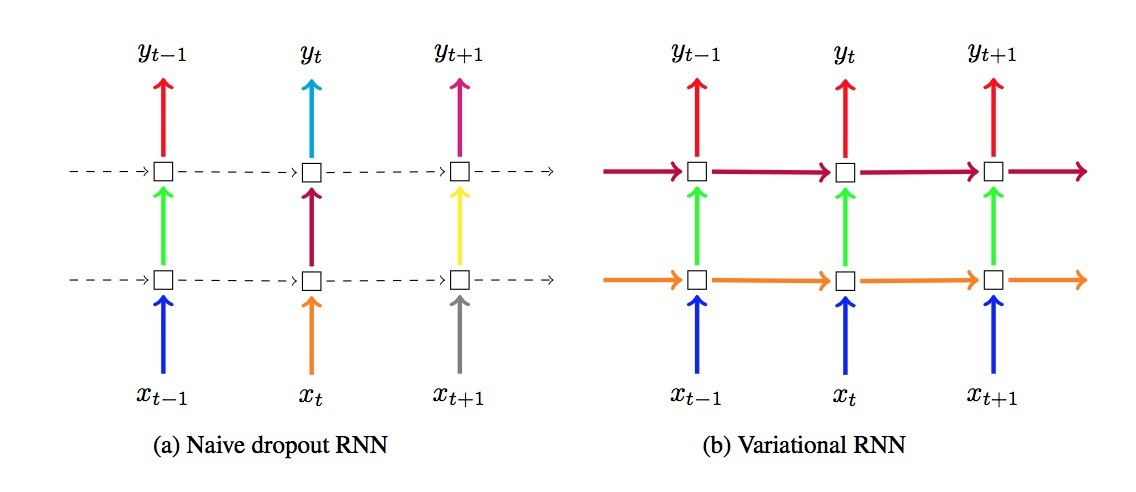摘要

RNN是深度学习许多研究的前研。这些模型的主要难点是容易overfit，直接在recurrent layers上应用dropout会失败。在Bayesian建模和深度学习的交叉研究上，提供了一种通用深度学习技术（比如：dropout）的Bayesian解释。在approximate Bayesian inference上的dropout基础，提供了一种理论扩展，并提供了在RNN模型上使用dropout的见解。我们在LSTM和GRU模型中基于dropout技术来使用这种新的变分推断（variantional inference），并将它应用于语言建模和语义分析任务上。新的方法要好于已存在的技术，并达到最好的效果。

1.介绍

RNN是基于序列的模型，是NLP、语言生成、视频处理、以及许多其它任务上的关键。模型的输入是一个符号序列，在每个timestep上，会将一个RNN unit应用于单个symbol，该网络的输出会使用来自之前的time step的信息。RNN很强，但很容易overfit。由于在RNN模型中缺乏正则化，使得它很难处理小数据，为了避免overfitting，研究者们通常会使用：early-stopping、或者小模型。3.背景

3.1 Bayesian神经网络

• 训练输入：$X = \lbrace x_1, \cdots, x_N\rbrace$
• 相应的输出：$Y = \lbrace y_1, \cdots, y_N\rbrace$

$p(y=d \mid x,w) = Categorical(\frac{exp(f_d^w(x))} { \sum\limits_{d'} exp(f_{d'}^w(x))})$

$p(y^* | x^*, X, Y) = \int p(y^*|x^*, w) p(w|X,Y) dw$

…(1)

3.2 Bayesian NN中的近似变分推断

$KL(q(w) || p(w|X,Y)) \propto \int q(w) log p(Y|X,w)dw + KL(q(w)||p(w)) \\ = -\sum\limits_1^N \int q(w) log p(y_i | f^w(x_i))dw + KL(q(w)||p(w))$

…(2)

4.RNN上的变分推断

$h_t = f_h(x_h, h_{t-1}) = \sigma(x_t W_h + h_{t-1} U_h + b_h)$

$\sigma$为非线性函数。该模型可以定义成：$f_y(h_T) = h_T W_y + b_y$。我们将该RNN看成是一个概率模型，将参数$w = \lbrace W_h,U_h,b_h,W_y,b_y \rbrace$看成是随机变量（遵循正态先验分布）。为了使在w上的依赖更清晰些，我们将$f_y$重写成$f_y^w$，同理$f_h^w$。我们定义了我们的概率模型的likelihood。在随机变量w的后验是相当复杂的，我们使用变分推断以及近似分布$q(w)$来近似它。

$\int q(w) log p(y|f_y^w(h_T)) dw = \int q(w) log p(y|f_y^w (f_h^w(X_T, h_{T-1}))) dw \\ = \int q(w) log p(y | f_y^w (f_h^w(x_T, f_h^w( \cdots f_h^w(x_1,h_0) \cdots )))) dw$

$\approx p(y | f_y^{\hat{w}} (f_h^{\hat{w}} (x_T, f_h^{\hat{w}}( \cdots f_h^{\hat{w}}(x_1,h_0) \cdots )))), \ \ \ \hat{w} \sim q(w)$

$L \approx -\sum\limits_{i=1}^N log p(y_i | f_y^{\hat{w}_i} f_h^{\hat{w}_i}(x_{i,T}, f_h^{\hat{w}_i}(x_{i,1},h_0) \cdots )))) + KL(q(w) || p(w))$

…(3)

$q(w_k) = p N(w_k; 0, \sigma^2 I) + (1-p) N(w_k; m_k, \sigma^2 I)$

• $m_k$是变分参数(row vector)
• p为(dropout probability)，事先给定
• $\sigma^2$较小

$p(y^* | x^*, X, Y) \approx \int p(y^*|x^*, w) q(w) d(w) \approx \frac{1}{K} \sum\limits_{k=1}^K p(y^* | x^*, \hat{w}_k)$

…(4)

4.1 在RNNs中dropout的实现与关系

$\underline{i} = sigm(h_{t-1} U_i + x_t W_i) \\ \underline{f} = sigm(h_{t-1} U_f + x_t W_f) \\ \underline{o} = sigm(h_{t-1} U_o + x_t W_o) \\ \underline{g} = sigm(h_{t-1} U_g + x_t W_g) \\ c_t = \underline{f} \circ c_{t-1} + \underline{i} \circ \underline{g} \\ h_t = \underline{o} \circ tanh(c_t)$

• $w = \lbrace W_i, U_i, W_f, U_f, W_o, U_o, W_g, U_g\rbrace$为权重矩阵
• $\circ$为element-wise product

$\begin{pmatrix} \underline{i} \\ \underline{f} \\ \underline{o} \\ \underline{g} \end{pmatrix} = \begin{pmatrix} sigm \\ sigm \\ sigm \\ tanh \end{pmatrix} ( \begin{pmatrix} x_t \\ h_{t-1} \end{pmatrix} ) \cdot W$

…(6)

$\begin{pmatrix} \underline{i} \\ \underline{f} \\ \underline{o} \\ \underline{g} \end{pmatrix} = \begin{pmatrix} sigm \\ sigm \\ sigm \\ tanh \end{pmatrix} ( \begin{pmatrix} x_t \circ z_x \\ h_{t-1} \circ z_h \end{pmatrix} \cdot W)$

…(7)

$\begin{pmatrix} \underline{i} \\ \underline{f} \\ \underline{o} \\ \underline{g} \end{pmatrix} = \begin{pmatrix} sigm \\ sigm \\ sigm \\ tanh \end{pmatrix} ( \begin{pmatrix} x_t \circ z_x^t \\ h_{t-1} \end{pmatrix} \cdot W)$

$c_t = c_t \circ z_c$

5.评估

#6.DropoutWrapper

参考

Updated on

STAR算法介绍

PLE介绍

Published on March 04, 2021

DTS介绍

Published on January 02, 2021